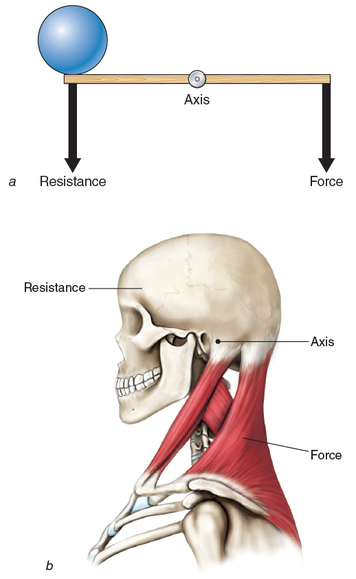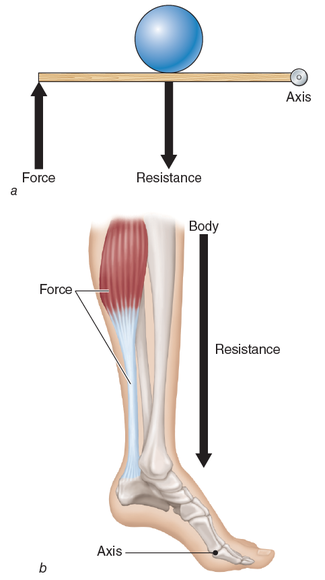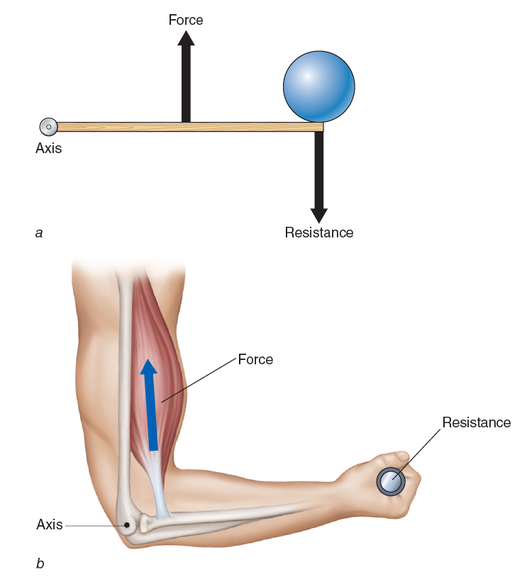Feedback

# Levers work to create movement in the human body

### This is an excerpt from Kinetic Anatomy w/Web Resource-3rd Edition by Robert Behnke.

Learn anatomy with practical exercises and activities in Kinetic Anatomy, Third Edition With Web Resource.

Bones, ligaments, and muscles are the structures that form levers in the body to create human movement. In simple terms, a joint (where two or more bones join together) forms the axis (or fulcrum), and the muscles crossing the joint apply the force to move a weight or resistance. Levers are typically labeled as first class, second class, or third class. All three types are found in the body, but most levers in the human body are third class.

A first-class lever has the axis (fulcrum) located between the weight (resistance) and the force (figure 1.21a). An example of a first-class lever is a pair of pliers or scissors. First-class levers in the human body are rare. One example is the joint between the head and the first vertebra (the atlantooccipital joint) (figure 1.21b). The weight (resistance) is the head, the axis is the joint, and the muscular action (force) come from any of the posterior muscles attaching to the skull, such as the trapezius.In a second-class lever, the weight (resistance) is located between the axis (fulcrum) and the force (figure 1.22a). The most obvious example is a wheelbarrow, where a weight is placed in the bed of the wheelbarrow between the wheel (axis) and the hands of the person using the wheelbarrow (force). In the human body, an example of a second-class lever is found in the lower leg when someone stands on tiptoes (figure 1.22b). The axis is formed by the metatarsophalangeal joints, the resistance is the weight of the body, and the force is applied to the calcaneus bone (heel) by the gastrocnemius and soleus muscles through the Achilles tendon.In a third-class lever, the most common in the human body, force is applied between the resistance (weight) and the axis (fulcrum) (figure 1.23a). Picture someone using a shovel to pick up an object. The axis is the end of the handle where the person grips with one hand. The other hand, placed somewhere along the shaft of the handle, applies force. At the other end of the shovel (the bed), a resistance (weight) is present. There are numerous third-class levers in the human body; one example can be illustrated in the elbow joint (figure 1.23b). The joint is the axis (fulcrum). The resistance (weight) is the forearm, wrist, and hand. The force is the biceps muscle when the elbow is flexed.